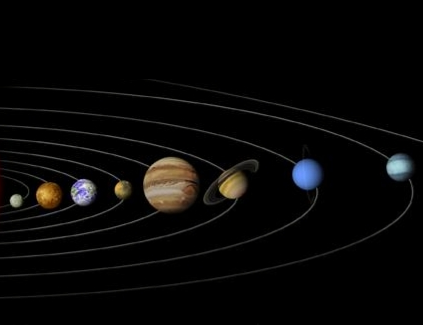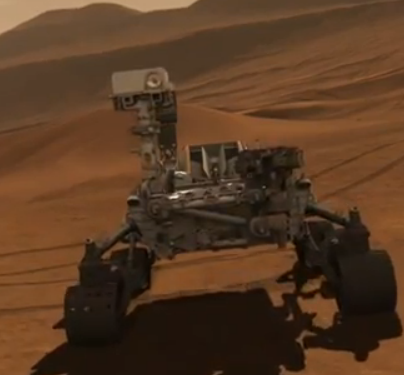﻿ Weight On Planets Calculator ﻿# Weight on Planets Calculator### How Much Would You Or Any Object Weigh On the Various Planets?Enter Weight: Any unit grams (g) kilograms (kg) pounds (lbs)

 Weight on Mercury: Weight on Mars: Weight on Uranus: Weight on Venus: Weight on Saturn: Weight on Neptune: Weight on Jupiter:

This Weight on Planets Conversion Calculator calculates your weight or the weight that any object would be if on the various planets in the solar system. This includes Mercury, Mars, Uranus, Venus, Neptune, Saturn, and Jupiter.

The list of the planets is in order of the least gravitational force (Mercury) to the greatest gravitational force (Jupiter).

To use this calculator, a user just enters his or her weight or the object's weight and clicks the 'Calculate' button, and the weight will automatically be computed and shown below. The weight can be entered in any units and the resultant answer will be in the same units which the user has input. Thus, for example, if a user enters 150lbs, the resultant answer of the weight in Planets would be 159.63lbs. The units will always match. Just for the sake of clarity, a user can select the units he wants the answer to appear in, whether grams, kilograms, or pounds, and that unit will show in the answer.

This calculator calculates the weight on Planets from the weight of the object on earth. Being that it takes the weight of an object on earth and converts it to the weight on Planets, the formula is Weight on Planets= (Weight on Earth/9.81m/s2) * gravitational force of the planet. To find the weight on Planets, we divide the weight on earth by the earth's force of gravity, which is 9.81m/s2. This calculates the mass of the object. Once we have the object's mass, we can find the weight by multiplying it by the gravitational force, which it is subject to.

Mercury has a gravitational force of 3.7m/s2.

Mars has a gravitational force of 3.711m/s2.

Uranus has a gravitational force of 8.69m/s2.

Venus has a gravitational force of 8.87m/s2.

Saturn has a gravitational force of 10.44m/s2.

Neptune has a gravitational force of 11.15m/s2.

Jupiter has a gravitational force of 24.79m/s2.

What causes the differences in weight between the various planets? The answer is the gravitational force which a planet is subject to. The difference gravitational forces are due to the different characteristics which make up the planet. Mass, density, and the diameter or size of the planet determines gravitational force. The larger the mass, density, and smaller the diameter of the planet, the greater the gravitational force will be. Conversely, the smaller the mass, density, and larger the diameter, the smaller the gravitational force.

Gravitational force is the downward force which is exerted on an object. This is why when you jump up into the air, you come back down. The Earth's gravitational force is pushing you back toward its surface. The greater the gravitational force, the more force is pushed down on you. Therefore, this translates into greater weight. Conversely, the less gravitational force there is, the less force there is pushing down on you. This translates into lesser weight. Objects on Mercury, which is the smallest planet in the solar system and has the least gravity, will weigh less than on any other planet. Objects on Jupiter, which is the largest planet and has the greatest gravitational force, will weigh the most out of any other planets.

Related Resources

﻿